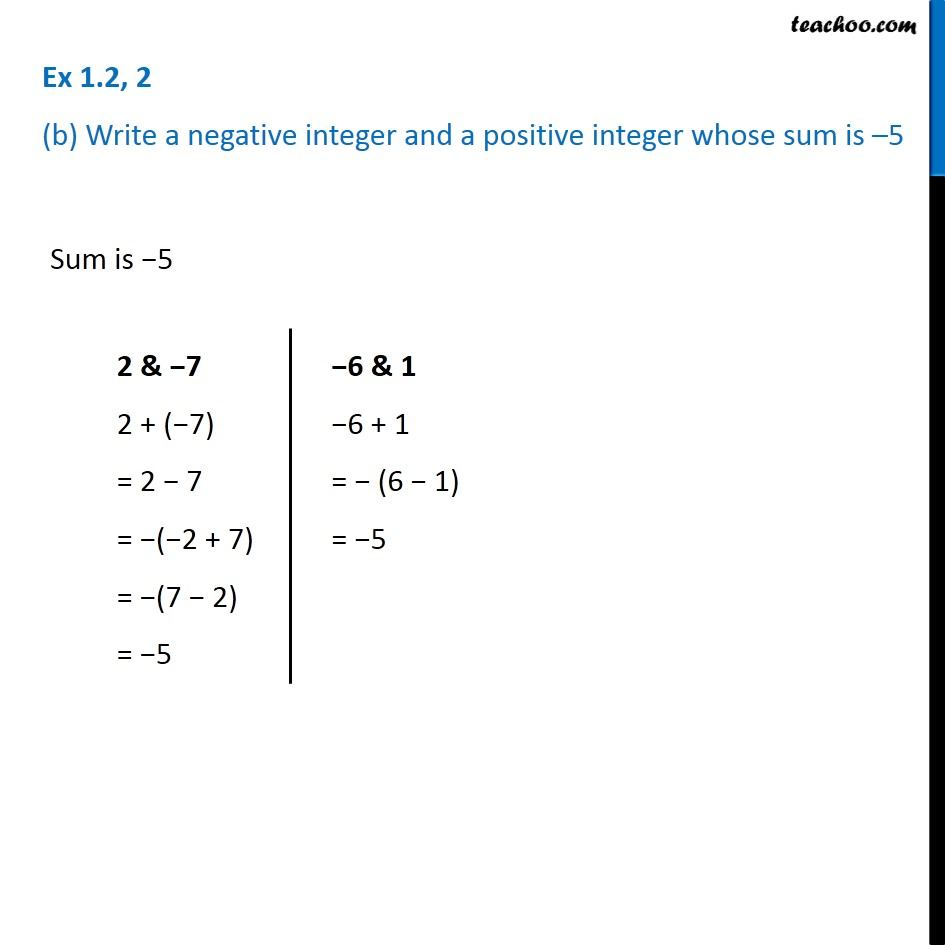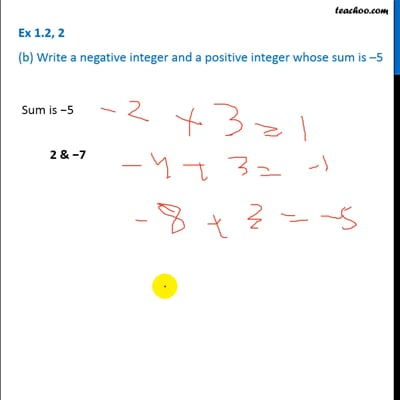Ex 1.2

Chapter 1 Class 7 Integers
Serial order wiseThis video is only available for Teachoo black users

Introducing your new favourite teacher - Teachoo Black, at only ₹83 per month

### Transcript

Ex 1.2, 2 (b) Write a negative integer and a positive integer whose sum is 5 Sum is 5 2 & 7 2 + ( 7) = 2 7 = ( 2 + 7) = (7 2) = 5 6 & 1 6 + 1 = (6 1) = 5